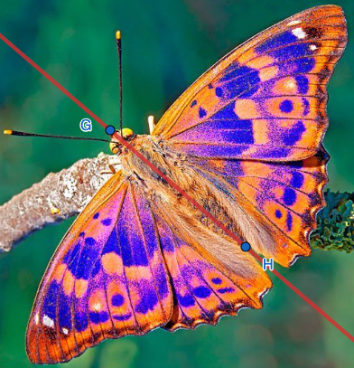# Axis of symmetry in the Spanish alphabet

Author:
Ana Megias

## What is an axis of symmetry?An axis of symmetry of a picture is the line that you can draw so that when you fold the picture through that line, both sides are a mirror image of the other one.A picture can have more than one axis of symmetry and they can go in any direction.

How many axes of symmetry can you find on letter A?

Select all that apply
• A
• B
• C
• D

How many axes of symmetry can you find on letter B?

Select all that apply
• A
• B
• C
• D

How many axes of symmetry can you find on letter C?

Select all that apply
• A
• B
• C
• D

How many axes of symmetry can you find on letter D?

Select all that apply
• A
• B
• C
• D

How many axes of symmetry can you find on letter E?

Select all that apply
• A
• B
• C
• D

How many axes of symmetry can you find on letter F?

Select all that apply
• A
• B
• C
• D

How many axes of symmetry can you find on letter G?

Select all that apply
• A
• B
• C
• D

How many axes of symmetry can you find on letter H?

Select all that apply
• A
• B
• C
• D

How many axes of symmetry can you find on letter I?

Select all that apply
• A
• B
• C
• D

How many axes of symmetry can you find on letter J?

Select all that apply
• A
• B
• C
• D

How many axes of symmetry can you find on letter K?

Select all that apply
• A
• B
• C
• D

How many axes of symmetry can you find on letter L?

Select all that apply
• A
• B
• C
• D

How many axes of symmetry can you find on letter M?

Select all that apply
• A
• B
• C
• D

How many axes of symmetry can you find on letter N?

Select all that apply
• A
• B
• C
• D

How many axes of symmetry can you find on letter Ñ?

Select all that apply
• A
• B
• C
• D

How many axes of symmetry can you find on letter O?

Select all that apply
• A
• B
• C
• D